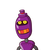# Q. a- (b x c) if a= 3, b= 6, c=9 Find the answer.

Q. a- (b x c)
if a= 3, b= 6, c=9

### 2 thoughts on “Q. a- (b x c)<br /> if a= 3, b= 6, c=9<br /> Find the answer.”

1.a-(b×c)

3-(6×9)

3-54

51

2.$$a – (b \times c) \\ = 3 – (6 \times 9) \\ = 3 – (54) \\ = 3 – 54 \\ = – 51$$